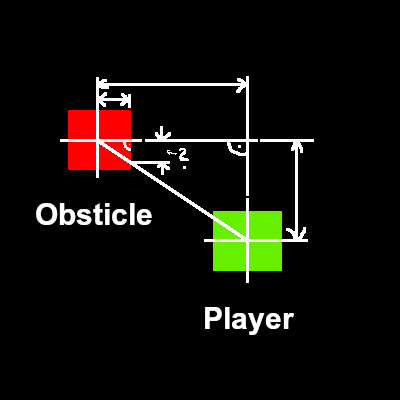# Mathquestion / dynamic light positionI have not done some trigonometry math in a long time.
i could figure this out using pytagoras, but i am not sure, if there might be a more efficient method to do so, or how the optimized formula would look like.

i did try to use raycasts and save the results in variables, but that did not work out (idk why).

Yes theorem of Pythagoras is one simple solution.
I suppose you know formula

i do.
my approach atm is i check in wich quadrant the player is and what distance to obsticle is greater (X or Y to determine whats a and whats b), then use pythagoras formula.

but i have the feeling there is a smarter and more efficient way.